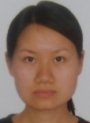Electron. J. Diff. Equ., Vol. 2012 (2012), No. 70, pp. 1-8.

### Uniqueness of positive solutions for a fractional differential equation via a fixed point theorem of a sum operator Chen Yang, Chengbo Zhai

Abstract:
In this work, we study the existence and uniqueness of positive solutions for nonlinear fractional differential equation boundary-value problems. Our analysis relies on a fixed point theorem of a sum operator. Our results guarantee the existence of a unique positive solution, and can be applied for constructing an iterative scheme for obtaining the solution.

Submitted January 27, 2012. Published May 7, 2012.
Math Subject Classifications: 34B18.
Key Words: Riemann-Liouville fractional derivative; positive solution; fractional differential equation; existence and uniqueness; fixed point theorem.

Show me the PDF file (192 KB), TEX file, and other files for this article.Chen Yang Department of Mathematics Business College of Shanxi University Taiyuan 030031 Shanxi, China email: yangchen0809@126.com Chengbo Zhai School of Mathematical Sciences, Shanxi University Taiyuan 030006 Shanxi, China email: cbzhai@sxu.edu.cn, cbzhai215@sohu.com